Berenger introduced the concept of a perfectly matched layer (PML) for reflectionless

absorption of electromagnetic waves, which can be employed as an alternative to the transparent boundary condition (TBC). The PML approach defines the truncation of the computation domain by layers (which absorb impinging plane waves) without any reflection, irrespective of their frequency and angle of incidence  – .

## PML equations

We consider a 3-D optical waveguide surrounded by PML regions I, II, and III with thickness d as shown in Figure 2. Using the transversely scaled version of PML, Maxwell’s equations can be written asFrom Equation 33 and Equation 34 we get: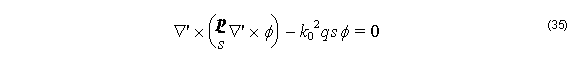with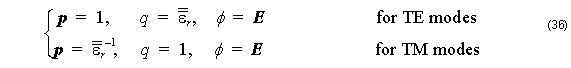whereHere E and H are the electric and magnetic field vectors, respectively, ω is the angular frequency, ε0  and μ0  are the permittivity and permeability of free space, respectively, n is the refractive index, and σe  and σm  are the electric and magnetic  conductivities of PML, respectively. The modified differential operator ∇’  used in Equation 33 and Equation 34 is defined as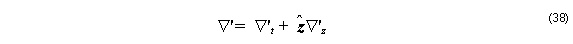withwhere xˆ , yˆ , and zˆ are the unit vectors in the x , y , and z directions, respectively, and the values of sx  are summarized in Table 1.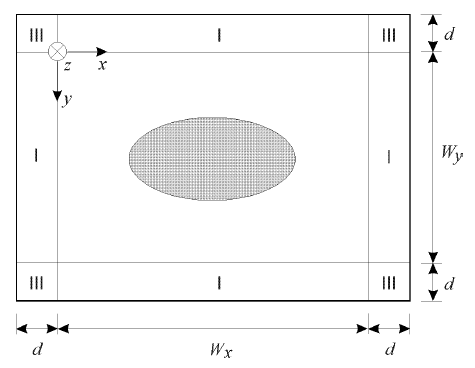Figure 2: Optical waveguide surrounded by PML

 Region sx sy I 1 s II s 1 III 1 1

Table 1:   Values of sx and sy

The relation in Equation 37 is required to satisfy the PML impedance matching conditionwhich means that wave impedance of PML medium exactly equals of the adjacent medium with refractive index n in the computation window,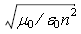, regardless of the angle of propagation or frequency.

In the PML medium, we assume an m th-power of the electric conductivity aswhere ρ is the distance from the beginning of PML. Using the theoretical reflection coefficient R at the interface between the computational window and the PML mediumthe maximum conductivity σmax may be determined as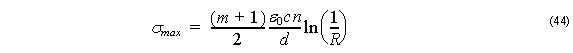where is the light velocity of free space.

The parameter s is written as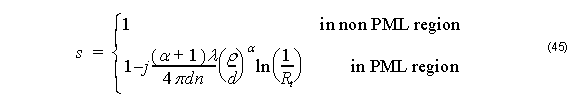where λ = 2πc ⁄ ω, d, x0, ρ are the free-space wavelength, the PML thickness, the position of the PML surface, and the theoretical reflection coefficient, respectively. Here, the PML is terminated with the perfect electric or magnetic conductor for TE or TM mode, respectively. Usually, a parabolic is assumed for the conductivity, =  2 .